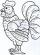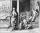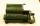# Dozen

What is the product of 26 and 5? Write the answer in Arabic numeral. Add up the digits. How many of this is in a dozen?
Divide #114 by this

Result

a =  130
b =  4
c =  3
d =  38

#### Solution:Leave us a comment of example and its solution (i.e. if it is still somewhat unclear...):

Showing 0 comments:Be the first to comment!## Next similar examples:

1. ProductResult of the product of the numbers 1, 2, 3, 1, 2, 0 is:
2. Roman numeralsWrite numbers written in Roman numerals as decimal.
3. Write decimalsWrite in the decimal system the short and advanced form of these numbers: a) four thousand seventy-nine b) five hundred and one thousand six hundred and ten c) nine million twenty-six
4. Addition of Roman numbersAdded together and write as decimal number: LXVII + MLXIV
5. RemaindersIt is given a set of numbers { 170; 244; 299; 333; 351; 391; 423; 644 }. Divide this numbers by number 66 and determine set of remainders. As result write sum of this remainders.
6. DigitsHow many odd four-digit numbers can we create from digits: 0, 3,5,6,7?
7. DivisionWhich number in division 16 give 12 and the rest 3?
8. The resultHow many times I decrease the number 1632 to get the result 24?
9. DoctorsIn the city operates 171 doctors. The city has 128934 citizens. How many citizens are per one doctor?
10. Math classificationIn 3A class are 27 students. One-third got a B in math and the rest got A. How many students received a B in math?
11. Divisibility 2How many divisors has integer number 13?
12. DivisibilityIs the number 761082 exactly divisible by 9? (the result is the integer and/or remainder is zero)
13. QuotientDetermine the quotient (q) and the remainder (r) from division numbers 100 and 8. Take the test of correctness.
14. ClassWhen Pythagoras asked how many students attend the school, said: "Half of the students studying mathematics, 1/4 music, seventh silent and there are three girls at school." How many students had Pythagoras at school?
15. Expression plus minusEvaluate expression: (-1)2 . 12 – 6 : 3 + (-3) . (-2) + 22 – (-3) . 2
16. NumberCalculate the integer number which, divided by 34 gives 10 and the rest 25.
17. IntegersMay be the sum of two integers less than their difference?